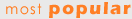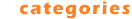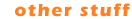Math Homework Maker : Software which can solve your math homework.
Math Homework Maker 1.0
 Quick Description: (Read All)Math Homework Maker,is a FREE software which can solve all your math homework Download Source: math.official.wsFile Ping Time (less is better): Could not ping file.

 Category: Education (Rank: 9 / 567): Mathematics (Rank: 2 / 93) Downloads: 2031 This Week (Rank: 204 / 15,688) / 2896 Total (Rank: 11,061 / 15,688) Developer: Naor Ben Meir Software Home Page: http://math-hw-maker.official.ws/ License Type: Freeware (\$0.00) Language: English Most Recent File Validation (?): Tue, February 5th, 2008 2:15 AM CST OS: Win95,Win98,WinME,WinXP,Windows2000,Windows2003 - Install and Uninstall. System Requirements: 300 MHz processor, 16 MB RAM

Full Description:
The Math Homework Maker, is a FREE software which can solve all your Math homework. If you are a pupil or a student, needing help with your math homework or just want to check them, Then you must have this software. The Math Homework Maker covers everything you need, from elementary school up to college. Among other things it covers: Conversion: Fraction, Improper Fractions, Decimal fractions. Operators On Fractions: [ add , subtraction, multiplication, division, bigger , equals ,smaller ] ,Common-Denominator, Reduction. Areas and Perimeters: Quadrilaterals, Triangles, Circles. Traffics and Percentages Problems. Solving Quadratic Equation. Progression: Geometric Progression, Arithmetic Progression. Triangles: The Law Of Sines, The Law Of Cosines. Statistics Mean, Standard-Deviation ,Variance. Complex Numbers. And Much More. This software is so easy and friendly to use even if you are a parent who want to check your kids' homework, but have no knowledge at all in mathematics.

Screenshot: (Source: www.angelfire.com - Ping: 105 ms - Size: 62 Kb (?)Keywords: math, mathematics, homework, student, pupil, algebra, education, equation, statistic, complex, numbers, progression, quadratic, fraction, parentRank Title - Education : Mathematics DownloadsCalc 3D (\$10) - Free calculator for vectors, matrices, complex numbers and quaternions 2048 2 Math Homework Maker (\$0) - Math Homework Maker,is a FREE software which can solve all your math homework 2031 3 MultyGraphiX (\$29.90) - MultyGraphix is the mathematical software designed to plot 2D and 3D functions 1994 4 GEUP (\$35) - Discover the power of Interactive Geometry for learning and doing Mathematics 1930 5 Linear Systems Beams (\$0) - Macintosh freeware for middle school mathmatics 1928Most Popular Editor's Choice Category Listing Education -Computer -Dictionaries -Geography -Kids -Languages -Mathematics -Other -Reference Tools -Science -TeachingSubmit Software Contact Us Terms of Use Other Sites Advertising